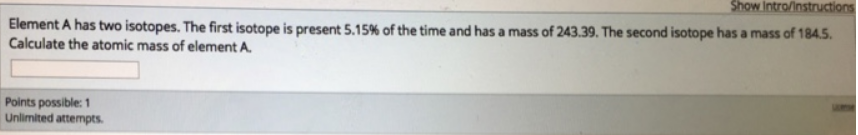Chemistry Isotopes Solution: Element A has two isotopes. The first isotope is p...

# Solution: Element A has two isotopes. The first isotope is present 5.15% of the time and has a mass of 243.39. The second isotope has a mass of 1845. Calculate the atomic mass of element A.

Problem

Element A has two isotopes. The first isotope is present 5.15% of the time and has a mass of 243.39. The second isotope has a mass of 1845. Calculate the atomic mass of element A.View Complete Written Solution